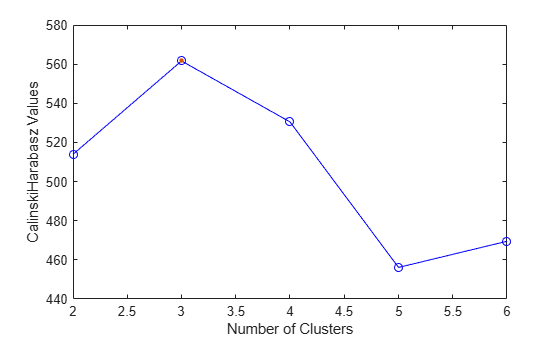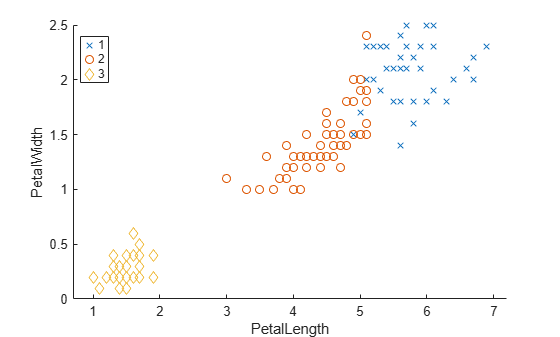# CalinskiHarabaszEvaluation

Calinski-Harabasz criterion clustering evaluation object

## Description

`CalinskiHarabaszEvaluation` is an object consisting of sample data (`X`), clustering data (`OptimalY`), and Calinski-Harabasz criterion values (`CriterionValues`) used to evaluate the optimal number of clusters (`OptimalK`). The Calinski-Harabasz criterion is sometimes called the variance ratio criterion (VRC). Well-defined clusters have a large between-cluster variance and a small within-cluster variance. The optimal number of clusters corresponds to the solution with the highest Calinski-Harabasz index value. For more information, see Calinski-Harabasz Criterion.

## Creation

Create a Calinski-Harabasz criterion clustering evaluation object by using the `evalclusters` function and specifying the criterion as `"CalinskiHarabasz"`.

You can then use `compact` to create a compact version of the Calinski-Harabasz criterion clustering evaluation object. The function removes the contents of the properties `X`, `OptimalY`, and `Missing`.

## Properties

expand all

### Clustering Evaluation Properties

Clustering algorithm used to cluster the sample data, returned as `'kmeans'`, `'linkage'`, `'gmdistribution'`, or a function handle. If you specify the clustering solutions as an input argument to `evalclusters` when you create the clustering evaluation object, then `ClusteringFunction` is empty.

ValueDescription
`'kmeans'`Cluster the data in `X` using the `kmeans` clustering algorithm, with `EmptyAction` set to `"singleton"` and `Replicates` set to `5`.
`'linkage'`Cluster the data in `X` using the `clusterdata` agglomerative clustering algorithm, with `Linkage` set to `"ward"`.
`'gmdistribution'`Cluster the data in `X` using the `gmdistribution` Gaussian mixture distribution algorithm, with `SharedCov` set to `true` and `Replicates` set to `5`.

Data Types: `double` | `char` | `function_handle`

Name of the criterion used for clustering evaluation, returned as `'CalinskiHarabasz'`.

Criterion values, returned as a numeric vector. Each value corresponds to a proposed number of clusters in `InspectedK`.

Data Types: `double`

List of the number of proposed clusters for which to compute criterion values, returned as a positive integer vector.

Data Types: `double`

Optimal number of clusters, returned as a positive integer scalar.

Data Types: `double`

Optimal clustering solution corresponding to `OptimalK`, returned as a positive integer column vector. Each row of `OptimalY` represents the cluster index of the corresponding observation (or row) in `X`. If you specify the clustering solutions as an input argument to `evalclusters` when you create the clustering evaluation object, or if the clustering evaluation object is compact (see `compact`), then `OptimalY` is empty.

Data Types: `double`

### Sample Data Properties

Excluded data, returned as a logical column vector. If an element of `Missing` is `true`, then the corresponding observation (or row) in the data matrix `X` is not used in the clustering solutions. If the clustering evaluation object is compact (see `compact`), then `Missing` is empty.

Data Types: `double` | `logical`

Number of observations in the data matrix `X`, ignoring observations with missing (`NaN`) values, returned as a positive integer scalar.

Data Types: `double`

Data used for clustering, returned as a numeric matrix. Rows correspond to observations, and columns correspond to variables. If the clustering evaluation object is compact (see `compact`), then `X` is empty.

Data Types: `single` | `double`

## Object Functions

 `addK` Evaluate additional numbers of clusters `compact` Compact clustering evaluation object `plot` Plot clustering evaluation object criterion values

## Examples

collapse all

Evaluate the optimal number of clusters using the Calinski-Harabasz clustering evaluation criterion.

Load the `fisheriris` data set. The data contains length and width measurements from the sepals and petals of three species of iris flowers.

`load fisheriris`

Evaluate the optimal number of clusters using the Calinski-Harabasz criterion. Cluster the data using `kmeans`.

```rng("default") % For reproducibility evaluation = evalclusters(meas,"kmeans","CalinskiHarabasz","KList",1:6)```
```evaluation = CalinskiHarabaszEvaluation with properties: NumObservations: 150 InspectedK: [1 2 3 4 5 6] CriterionValues: [NaN 513.9245 561.6278 530.4871 456.1279 469.5068] OptimalK: 3 ```

The `OptimalK` value indicates that, based on the Calinski-Harabasz criterion, the optimal number of clusters is three.

Plot the Calinski-Harabasz criterion values for each number of clusters tested.

`plot(evaluation)`The plot shows that the highest Calinski-Harabasz value occurs at three clusters, suggesting that the optimal number of clusters is three.

Create a grouped scatter plot to examine the relationship between petal length and width. Group the data by suggested clusters.

```PetalLength = meas(:,3); PetalWidth = meas(:,4); clusters = evaluation.OptimalY; gscatter(PetalLength,PetalWidth,clusters,[],"xod");```The plot shows cluster 3 in the lower-left corner, completely separated from the other two clusters. Cluster 3 contains flowers with the smallest petal widths and lengths. Cluster 1 is in the upper-right corner, and contains flowers with the largest petal widths and lengths. Cluster 2 is near the center of the plot, and contains flowers with measurements between these two extremes.